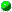`All Packages  Class Hierarchy  This Package  Previous  Next  Index`

# Class juice.vector.RealSpc

```java.lang.Object
|
+----juice.vector.RealSpc
```

public class RealSpc
extends Object
implements RealSpace
This class has no public constructors. Use the static method getSpace() to get an instance of RealSpc.

##copy()
Since there is at most only one instance of a RealSpc, this must be a shallow copy.equals(Object)
Compares two Objects for equality.getSpace()
This acts as the constructor for RealSpc.
Because every instance of RealSpc represents the same mathematical space, it makes sense to allow at most one instance of RealSpc to be in existence at a time.
This static method can enforce that rule.hasMember(Vector)newMember()toString()
Returns a string representation of the object.

##getSpace
``` public static RealSpc getSpace()
```
This acts as the constructor for RealSpc.
Because every instance of RealSpc represents the same mathematical space, it makes sense to allow at most one instance of RealSpc to be in existence at a time.
This static method can enforce that rule.newMember
``` public Vector newMember()
```hasMember
``` public boolean hasMember(Vector v)
```copy
``` public JamObject copy()
```
Since there is at most only one instance of a RealSpc, this must be a shallow copy.equals
``` public boolean equals(Object obj)
```
Compares two Objects for equality.

Overrides:
equals in class ObjecttoString
``` public String toString()
```
Returns a string representation of the object.

Overrides:
toString in class Object

`All Packages  Class Hierarchy  This Package  Previous  Next  Index`